# Mechanics

2 posts / 0 new
Gerard N GodwinMechanics

From the circular disk of diameter 100mm is cut out a circle whose diameter is the radius of the disk. F ind the center of gravity of remaining portion.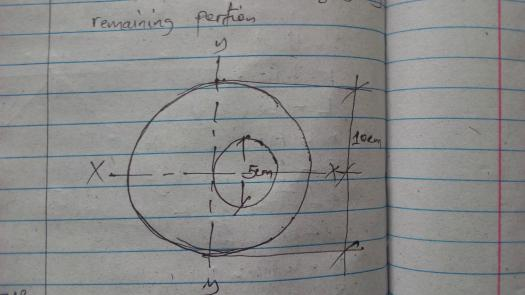fitzmerl duronAh! You mean this?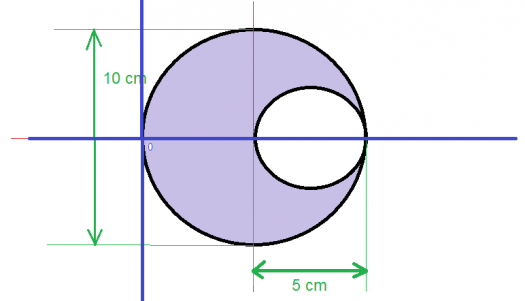To get the coordinates of centroid of the figure above, we need to use the formula

$$A \bar x = A_1x_1 + A_2x_2 + A_3x_3 +A_4x_4 +....A_n x_n$$ $$A \bar y = A_1y_1 + A_2y_2 + A_3y_3 +A_4y_4 +....A_n y_n$$

where:

$A =$ area of the whole figure

$x =$ distance of the centroid from the $x$-axis

$A_1 =$ area of one part of the figure

$x_1 =$ distance of its particular centroid from the $x$-axis

.

.

.

.

.

.

$y =$ distance of the centroid from the $y$-axis

$A_1 =$ area of one part of the figure

$y_1 =$ distance of its particular centroid from the $y$-axis

The formula that is applicable to the figure above would be:

$$A \bar x = A_1x_1 + A_2x_2 + A_3x_3 +A_4x_4$$ $$A \bar y = A_1y_1 + A_2y_2 + A_3y_3 +A_4y_4$$

Redrawing the figure to make things clearer: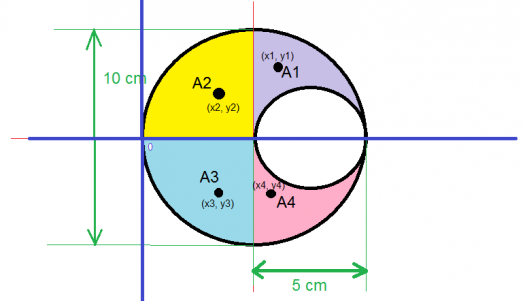Now getting the A, A$_1$, A$_2$, A$_3$, A$_4$:

For A:

$$A = \pi R^2 - \pi r^2$$ $$A = \pi (5 \space cm)^2 - \pi (2.5 \space cm)^2$$ $$A = 25\pi \space cm^2 - 6.25\pi \space cm^2$$ $$A = 18.75\pi \space cm^2$$

For A$_1$:

$$A_1 = \frac{25}{4}\pi \space cm^2 - \frac{6.25}{2}\pi\space cm^2$$ $$A_1 = 3.125\pi \space cm^2$$

For A$_4$:

$$A_4 = \frac{25}{4}\pi \space cm^2 - \frac{6.25}{2}\pi\space cm^2$$ $$A_4 = 3.125\pi \space cm^2$$

For A$_2$:

$$A_2 = \frac{25}{4}\pi \space cm^2$$

For A$_3$:

$$A_3 = \frac{25}{4}\pi \space cm^2$$

Now getting the x, x$_1$, x$_2$, x$_3$, x$_4$:

For x:

We can get it after I got x$_1$, x$_2$, x$_3$, x$_4$

For x$_1$: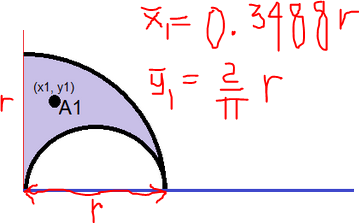Then the centroidal coordinates of A$_1$ is:

$$x_1 = 0.3488 r$$ $$x_1 = (0.3488) (5 \space cm)$$ $$x_1 = 1.744 \space cm$$

The real value of $\bar x$ is:

$$\bar x_1 = 5 + 1.744 \space cm = 6.744 \space cm$$

For x$_2$

The centroidal coordinates of a quarter circle is: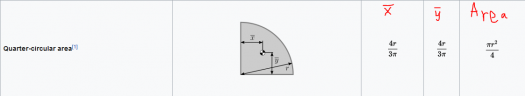$$\bar x = \frac{4}{3\pi} r$$ $$\bar y = \frac{4}{3\pi} r$$

Then the centroidal coordinates of A$_2$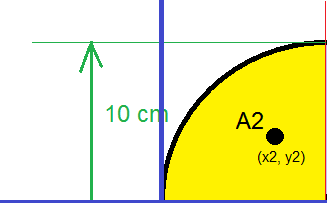$$x_2 = \frac{4}{3\pi} r$$ $$x_2 = \frac{4}{3\pi} (5 \space cm)$$ $$x_2 = 2.1 \space cm$$

The real value of $x_2$ is:

$$x_3 = 5-2.1 \space cm = 2.9 \space cm$$

For x$_3$:

The centroidal coordinates of a quarter circle is:$$x = \frac{4}{3\pi} r$$ $$y = \frac{4}{3\pi} r$$

Then the centroidal coordinates of A$_3$$$x_3 = \frac{4}{3\pi} r$$ $$x_3 = \frac{4}{3\pi} (5 \space cm)$$ $$x_3 = 2.1 \space cm$$

The real value of $x_3$ is:

$$x_3 = 5-2.1 \space cm = 2.9 \space cm$$

For x$_4$:Then the centroidal coordinates of A$_4$ is:

$$x_4 = 0.3488 r$$ $$x_4 = (0.3488) (5 \space cm)$$ $$x_4 = 1.744 \space cm$$

The real value of $x_4$ is:

$$x_4 = 5 + 1.744 \space cm = 6.74 \space cm$$

We can now get the $\bar x$:

$$A \bar x = A_1x_1 + A_2x_2 + A_3x_3 +A_4x_4$$ $$(18.75\pi \space cm^2) \bar x = (3.125\pi \space cm^2)(6.744 \space cm) + (\frac{25}{4}\pi \space cm^2)(2.9 \space cm) + (\frac{25}{4}\pi \space cm^2)(2.9 \space cm) + (3.125\pi \space cm^2)(6.744 \space cm)$$ $$\bar x = 4.18 \space cm$$

To get the $\bar y$, notice that the figure is symmetrical, so the $\bar y$ centroidal coordinate would be $0$

Therefore, the centroid $C(\bar x, \bar y)$ of the figure above would be $C(\bar x, \bar y) = C(4.18, 0)$

Alternate solutions are encouraged:-)

• Mathematics inside the configured delimiters is rendered by MathJax. The default math delimiters are $$...$$ and $...$ for displayed mathematics, and $...$ and $...$ for in-line mathematics.